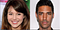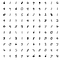# A Brief Introduction To GANs

## With explanations of the math and code

GANs, or Generative Adversarial Networks, are a type of neural network architecture that allow neural networks to generate data. In the past few years, they’ve become one of the hottest subfields in deep learning, going from generating fuzzy images of digits to photorealistic images of faces.Before: fuzzy digits, After: photorealistic faces

Variants of GANs have now done insane stuff, like converting images of zebras to horses and vice versa.

I found GANs fascinating, and in an effort to understand them better, I thought that I’d write this article, and in the process of explaining the math and code behind them, understand them better myself.

Here’s a link to a github repo I made for GAN resources:

# So how do GANs work?

GANs learn a probability distribution of a dataset by pitting two neural networks against each other.

Here’s a great article that explains probability distributions and other concepts for those who aren’t familiar with them:

One model, the generator, acts akin to a painting forger. It tries to create images that look very similar to the dataset. The other model, the discriminator, acts like the police, and tries to detect whether the images generated were fake or not.

What basically happens, is that the forger keeps getting better at making fakes, while the police keep getting better at detecting fakes. Effectively, these two models keep trying to beat each other, until after many iterations, the generator creates images indistinguishable from the real dataset.

Training generative adversarial networks involve two objectives:

1. The discriminator maximizes the probability of assigning the correct label to both training examples and images generated by the generator. I.e the policeman becomes better at differentiating between fakes and real paintings.
2. The generator minimizes the probability that the discriminator can predict that what it generates is fake. I.e the generator becomes better at creating fakes

Let’s try and encode these two ideas into a program.

We’ll be following this code in this tutorial

# The Data

GANs need a dataset to use, so for this tutorial, we’ll be using the classic hello world to machine learning — MNIST, a dataset of handwritten digits.

The generator also needs random input vectors to generate images, and for this, we’ll be using numpy

# The GAN Function

The GAN plays a minimax game, where the entire network attempts to optimize the function V(D,G). This is the equation that defines what a GAN is doing:

Now to anyone who isn’t well versed in the math behind it, it looks terrifying, but the idea it represents is simple, yet powerful. It’s just a mathematical representation of the two objectives as defined above.

The generator is defined by G(z), which converts some noise z we input into some data, like images.

The discriminator is defined by D(x), which outputs the probability that the input x came from the real dataset or not.The discriminator acts like the police

We want the predictions on the dataset by the discriminator to be as close to 1 as possible, and on the generator to be as close to 0 as possible. To achieve this, we use the log-likelihood of D(x) and 1-D(z) in the objective function.

The log just makes sure that the closer it is to an incorrect value, the more it is penalized.

Here’s an explanation for log loss if you aren’t sure what it does:

## Coding the Generator

The generator is just a vanilla neural network model that takes a random input vector and outputs a 784-dim vector, which, when reshaped, becomes a 28*28 pixel image.

## Coding the Discriminator

The discriminator is another neural network that takes the output of the previous network, a 784-dimensional vector, and outputs a probability between 0 and 1 that it came from the training dataset.

## Compiling it into a GAN

We now compile both models into a single adversarial network, setting the input as a 100-dimensional vector, and the output as the output of the discriminator.

## Training the GAN

1. First, we load the data and split the data into several batches to feed into our model
2. Here we just initialize our GAN network based on the methods defined above
3. This is our training loop, where we run for the specified number of epochs.
4. We generate some random noise and take out some images from our dataset
5. We generate some images using the generator and create a vector X that has some fake images and some real images
6. We create a vector Y which has the “correct answers” that corresponds to X, with the fake images labeled 0 and the real images labeled 0.9. They’re labeled 0.9 instead of 1 because it helps the GAN train better, a method called one-sided label smoothing.
7. We need to alternate the training between the discriminator and generator, so over here, we update the discriminator
8. Finally, we update the discriminator.

# Our first GAN

Once we run the code above, we’ve effectively created our first GAN that generates digits from scratch!Images generated from the GAN we trained!

Hopefully, this article provided an introduction to Generative Adversarial Networks and how to make one. In the near future, I’ll be writing a lot more about machine learning, do keep updated!

Thanks for reading,

Sarvasv

934 claps

Written by

## Sarvasv Kulpati

Writing about technology, philosophy, and everything in between.

## Sigmoid

Making Machine Learning more accessible. One line of code at a time.